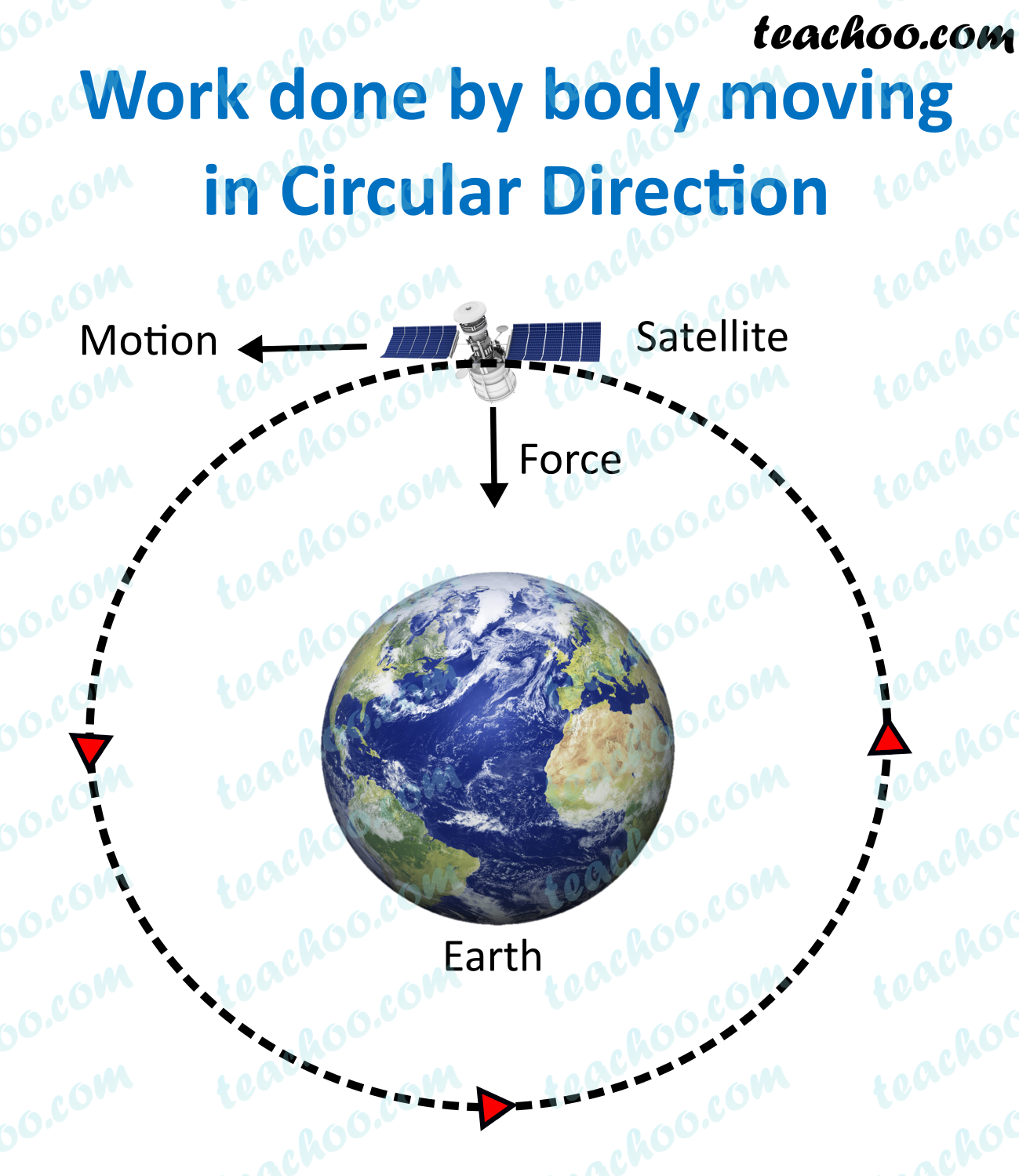Concepts

Class 9
Chapter 11 Class 9 - Work and EnergyWhen a body moves in circular direction

The direction of force is towards the radius

And direction of displacement is along the tangent

Since tangent is always perpendicular to radius

Hence, direction of force and displacement is always 90 degree

So, Work Done is 0

## Explanation

Work = Force × Distance × cos θ

Work = Force × Distance × cos 90

Since cos 90 degree = 0

Work = Force × Distance × 0

Work = 0

Hence, we can say that work done by object in moving cirular direction is 0

Example

Work done by earth in revolving around sun is 0

Work done by satellite moving around earth is always 0

## Is motion of earth around sun postive, negative or zero work?

Earth revolves around sun in an almost circular orbit

Force experienced is gravitational force, which is towards the center along the radius

And displacement is along the tangent.

Since tangent is always perpendicular to the radius

Therefore, angle between force and the displacement is 90 degrees.

Hence, no work is done

## Questions

NCERT Question 11 - What is the work done by the force of gravity on a satellite moving round the earth? Justify your answer.

Learn in your speed, with individual attention - Teachoo Maths 1-on-1 Class